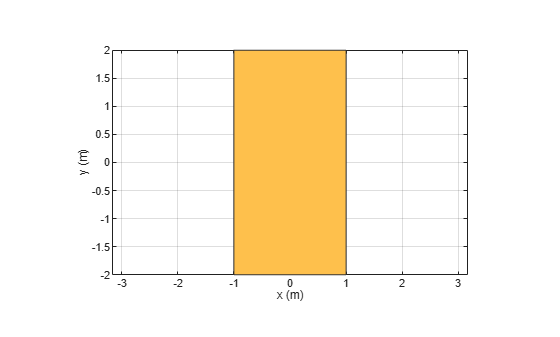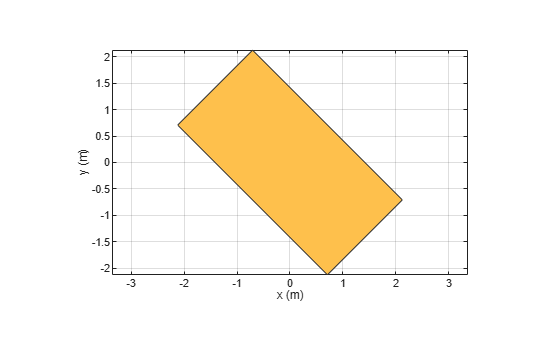# rotateZ

Rotate shape about z-axis and angle

## Syntax

``rotateZ(shape,angle)``
``c = rotateZ(shape,angle)``

## Description

example

````rotateZ(shape,angle)` rotate shape about the z-axis and angle.```
````c = rotateZ(shape,angle)` rotate shape about the z-axis and angle.```

## Examples

collapse all

Create and view a rectangle with a length of 2 m and a width of 4 m.

```r2 = antenna.Rectangle('Length',2,'Width',4); show(r2) axis equal ```Rotate the rectangle.

```rotateZ(r2,45); show(r2) ```## Input Arguments

collapse all

Shape created using custom elements and shape objects of Antenna Toolbox, specified as an object.

Example: `rotateZ(rectangle)` where rectangle is created using `antenna.Rectangle` object.

Angle of rotation, specified as a scalar in degrees.

Example: `rotateZ(rectangle,45)` rotates the rectangle around z-axis by 45 degrees.

Data Types: `double`